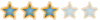Montreal Math ClubMathematics students in Montreal - Étudiants de mathématiques à MontréalHomeCalendarFAQSearchMemberlistUsergroupsRegisterLog in

 Montreal Math Club :: Mathematics :: Problem Solving :: Solved problems Share

# [SOLVED] Sum of periodic functionsAuthorMessage

AdminPosts : 184
Join date : 2009-09-15
Age : 32
Location : the infinite, frictionless plane of uniform densitySubject: [SOLVED] Sum of periodic functionsSun Nov 08, 2009 9:18 pm Let m(x) and n(x) be two continuous functions R-->R. Suppose m(x) is periodic with period M and n(x) is periodic with period N. Show that m(x)+n(x) is periodic if and only if M/N is rational.Last edited by Bruno on Wed Nov 11, 2009 5:51 pm; edited 1 time in totalDescartesPosts : 100
Join date : 2009-11-05
Age : 35
Location : Right behind youSubject: Re: [SOLVED] Sum of periodic functionsSun Nov 08, 2009 9:54 pm Sol: simply solvedif M/N be an irrational number then the set {mM-nN where m,n\in \mathbb{N}} is dense in \mathbb{R}, so you then get functions m and n just differs by a constant and more M=N which is of course a contradiction.AdminPosts : 184
Join date : 2009-09-15
Age : 32
Location : the infinite, frictionless plane of uniform densitySubject: Re: [SOLVED] Sum of periodic functionsSun Nov 08, 2009 10:15 pm It's good, I think you have the general idea, but you don't give much details!Where do you use the continuity of m and n?DescartesPosts : 100
Join date : 2009-11-05
Age : 35
Location : Right behind youSubject: Re: [SOLVED] Sum of periodic functionsSun Nov 08, 2009 10:21 pm suppose that m_kM-n_kN is convergent to x_0 so you get m(x_0)+n(x_0)= lim {m(m_kM-n_kN)+n(m_kM-n_kN)}=lim{m(-n_kN)+n(m_kM)}=m(\infty)+n(\infty)=const.DescartesPosts : 100
Join date : 2009-11-05
Age : 35
Location : Right behind youSubject: Re: [SOLVED] Sum of periodic functionsSun Nov 08, 2009 10:27 pm, by the way, your proof today was just awesomeSponsored contentSubject: Re: [SOLVED] Sum of periodic functions[SOLVED] Sum of periodic functionsPage 1 of 1
 Similar topics» solved scanner

Permissions in this forum:You cannot reply to topics in this forumJump to: Select a forum||--Math Club|   |--Mathematical Sundays|   |--Suggestions|   |--Introduce yourself|   |--Mathematics|   |--Problem Solving|   |   |--Solved problems|   |   |   |--Calculus and Analysis|   |--Combinatorics|   |--Geometry|   |--Number Theory|   |--Probabilities and statistics|   |--Set theory and Topology|   |--Online Resources|   |--Chit chat    |--The Water Cooler    |--Mathematical poems